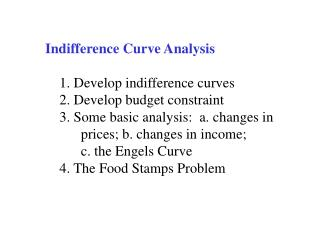# Indifference Curve Analysis 1. Develop indifference curves 2. Develop budget constraint 3. Some basic analysis: - PowerPoint PPT PresentationDownload PresentationIndifference Curve Analysis 1. Develop indifference curves 2. Develop budget constraint 3. Some basic analysis:

Indifference Curve Analysis 1. Develop indifference curves 2. Develop budget constraint 3. Some basic analysis:
Download Presentation## Indifference Curve Analysis 1. Develop indifference curves 2. Develop budget constraint 3. Some basic analysis:

- - - - - - - - - - - - - - - - - - - - - - - - - - - E N D - - - - - - - - - - - - - - - - - - - - - - - - - - -
##### Presentation Transcript

1. Indifference Curve Analysis 1. Develop indifference curves 2. Develop budget constraint 3. Some basic analysis: a. changes in prices; b. changes in income; c. the Engels Curve 4. The Food Stamps Problem

2. Indifference curve: A collection of points for which the consumer is indifference between each of them and some reference point. Typically shown in the context of a two good world on a two-dimensional graph.

3. The axiomatic approach to indifference • curves is a search for a minimum set • of assumptions regarding consumer • behavior through which to generate • indifference curves. • Standard axioms: • More is preferred to less—nonsatiation • Completeness—all points in a relation • Transitivity– A  B; B  C;  A  C

4. The budget equation: • B = pogOG + pfF • OG = B/pog – pf/pogF Meaning: The budget equation will depict a curve in OG-Food space that is downward sloping (note: its derivative –pf/pog is negative).

5. Applying calculus to find an expression for the slope of the other curve, the indifference curve: OG/F = - (U/F) /(U/OG) or, using an equivalent notation: OG/F = - MUf/MUog

6. At an equilibrium, tangency implies that the slope of the budget constraint equals the slope of the indifference curve: Hence,

7. Consumer equilibrium requires that pf/pog = MUf /MUog or MUf /pf = MUog /pog In words: The marginal utility per dollar of expenditure must be equal for each good.